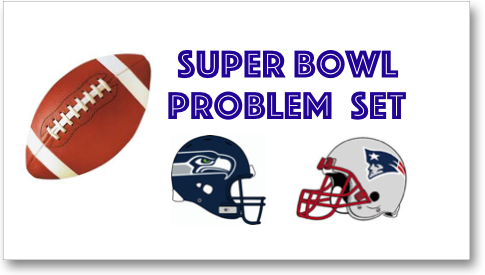# Super Bowl Problem Set

By Renee | Added Jan 28, 2015NFL Super Bowl XLIX is coming up this Sunday, so it’s time to warm up with some football themed math problems!

### Lower Elementary:

Question: Kelsey bought a pack of 92 foam fingers to root for the New England Patriots. She passed out half of them at the Patriots tailgating party before the Super Bowl. She then passed out half of the remaining foam fingers during the game to fans in the stands. How many foam fingers does Kelsey have left?

Solution: Half of 92 is the same as half of 90 + half of 2. Half of 90 is 45 and half of 2 is 1. 45 + 1 = 46. Half of 92 is 46. Kelsey passed out 46 foam fingers during the tailgating party. Then she passed out half of the remaining amount foam fingers during the game. Half of 46 is the same as half of 40 + half of 6. Half of 40 is 20 and half of 6 is 3. 20 + 3 = 23. She passed out 23 foam fingers during the game, which means that she has 23 foam fingers left.

### Upper Elementary:

Question: Seattle Seahawks quarterback Russell Wilson is exercising to prepare for the big game this Sunday. He starts by doing 5 sets of 15 push-ups. After doing other exercises, he cools down by doing an additional 10 push-ups. How many total push-ups did Russell do?

Solution: To see how many push-ups Russell did before the rest of his routine, multiply 15 by 5. 15 × 5 = 75. He does 10 push-ups to cool down, so add 10 to get the total number of push-ups Russell does. 75 + 10 = 85 push-ups.

### Middle School:

Question: Alexis, a Seattle Seahawks fan, runs at an average speed of 5 feet per second. How many minutes will it take for her to run the length of a football field (100 yards) twice before the big game?

Solution: To find how long it will take, we need the units to have the same name: her speed is in feet per second and the distance is in yards. One way is to convert the yards to feet by multiplying by 3 because there are 3 feet in 1 yard. 100 × 3 = 300. She runs the length of the football field twice, so we multiply this by 2. 300 x 2= 600. So now we calculate how long it would take her to run 600 feet. To see how long it takes, divide the distance traveled by the rate at which Alexis runs. 600 ÷ 5 = 120 seconds. It takes Alexis 120 seconds to run the length of the field twice. To convert seconds to minutes, divide by 60 because there are 60 seconds in 1 minute. 120 ÷ 60 = 2. It would take Alexis 2 minutes to run the length of the football field twice.

### Algebra and Up:

Question: New England Patriots punter Ryan Allen kicked the ball from the end zone. The ball followed a path that is modeled by the function f(t) = -3t^2 + 24t, where t is time in seconds and f(t) is the how high the ball is off the ground in yards. How long will it take the ball to hit the ground?

Solution: To find when the ball will hit the ground, we have to set f(t) = 0, because that is when the ball will hit the ground. So our problem becomes

0 = -3t^2 + 24t

One way to solve this problem is to factor the equation. Each term has a 3t in common, so we can factor that out.

0 = 3t(-t + 8)

By the zero product property, if two terms multiply to equal zero, then one of the terms must also equal zero. So, set each term equal to 0.

3t = 0

Divide by 3.

t = 0

Now for the other term.

-t + 8 = 0

Subtract 8 from both sides.

-t = -8

Multiply both sides by -1.

t = 8.

So the ball is on the ground when t = 0 and t = 8. Ryan kicks the ball when t = 0 because that is when we start counting how long the ball is in the air. That means that the ball hits the ground at t = 8, or 8 seconds from when Ryan kicked the ball.